1. /
2. CBSE
3. /
4. Class 07
5. /
6. Mathematics
7. /
8. NCERT Solutions for Class...

# NCERT Solutions for Class 7 Maths Exercise 7.2### myCBSEguide App

Download the app to get CBSE Sample Papers 2023-24, NCERT Solutions (Revised), Most Important Questions, Previous Year Question Bank, Mock Tests, and Detailed Notes.

NCERT solutions for Maths Congruence of Triangles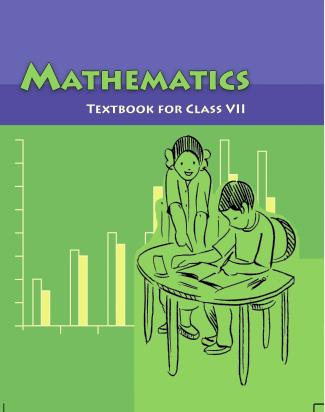## NCERT Solutions for Class 7 Maths Congruence of Triangles

###### Question 1.Which congruence criterion do you use in the following?

(a) Given: AC = DF, AB = DE, BC = EF

So {tex}\Delta {/tex}ABC {tex} \cong \Delta {/tex}DEF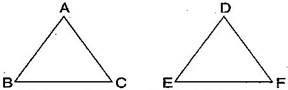(b) Given: RP = ZX, RQ = ZY, {tex}\angle {/tex}PRQ = {tex}\angle {/tex}XZY

So {tex}\Delta {/tex}PQR {tex} \cong \Delta {/tex}XYZ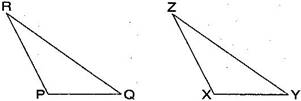(c) Given: {tex}\angle {/tex}MLN = {tex}\angle {/tex}FGH, {tex}\angle {/tex}NML = {tex}\angle {/tex}HFG, ML = FG

So {tex}\Delta {/tex}LMN {tex} \cong \Delta {/tex}GFH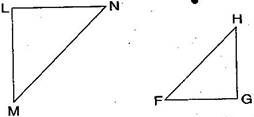(d) Given: EB = BD, AE = CB, {tex}\angle {/tex}A = {tex}\angle {/tex}C = {tex}{90^ \circ }{/tex}

So {tex}\Delta {/tex}ABE {tex} \cong \Delta {/tex}CDB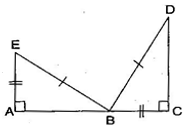(a) By SSS congruence criterion, since it is given that AC = DF, AB = DE, BC = EF

The three sides of one triangle are equal to the three corresponding sides of another triangle.

Therefore, {tex}\Delta {/tex}ABC {tex} \cong {/tex}{tex}\Delta {/tex}DEF

(b) By SAS congruence criterion, since it is given that RP = ZX, RQ = ZY and {tex}\angle {/tex}PRQ = {tex}\angle {/tex}XZY

The two sides and one angle in one of the triangle are equal to the corresponding sides and the angle of other triangle.

Therefore, {tex}\Delta {/tex}PQR {tex} \cong {/tex}{tex}\Delta {/tex}XYZ

(c) By ASA congruence criterion, since it is given that {tex}\angle {/tex}MLN = {tex}\angle {/tex}FGH, {tex}\angle {/tex}NML = {tex}\angle {/tex}HFG, ML = FG.

The two angles and one side in one of the triangle are equal to the corresponding angles and side of other triangle.

Therefore, {tex}\Delta {/tex}LMN {tex} \cong {/tex}{tex}\Delta {/tex}GFH

(d) By RHS congruence criterion, since it is given that EB = BD, AE = CB, {tex}\angle {/tex}A = {tex}\angle {/tex}C = {tex}{90^ \circ }{/tex}

Hypotenuse and one side of a right angled triangle are respectively equal to the hypotenuse and one side of another right angled triangle.

Therefore, {tex}\Delta {/tex}ABE {tex} \cong {/tex}{tex}\Delta {/tex}CDB

NCERT Solutions for Class 7 Maths Exercise 7.2

###### Question 2.You want to show that {tex}\Delta {/tex}ART {tex} \cong \Delta {/tex}PEN:
1. If you have to use SSS criterion, then you need to show:

(i) AR = (ii) RT = (iii) AT =

1. If it is given that {tex}\angle {/tex}T = {tex}\angle {/tex}N and you are to use SAS criterion, you need to have:

(i) RT = and(ii) PN =

1. If it is given that AT = PN and you are to use ASA criterion, you need to have:

(i) ? (ii) ?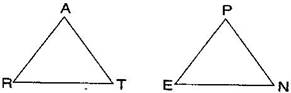(a) Using SSS criterion, {tex}\Delta {/tex}ART {tex} \cong {/tex}{tex}\Delta {/tex}PEN

(i) AR = PE (ii) RT = EN (iii) AT = PN

(b) Given: {tex}\angle {/tex}T = {tex}\angle {/tex}N

Using SAS criterion, {tex}\Delta {/tex}ART {tex} \cong {/tex}{tex}\Delta {/tex}PEN

(i) RT = EN (ii) PN = AT

(c) Given: AT = PN

Using ASA criterion, {tex}\Delta {/tex}ART {tex} \cong {/tex}{tex}\Delta {/tex}PEN

(i) {tex}\angle {/tex}RAT = {tex}\angle {/tex}EPN (ii) {tex}\angle {/tex}RTA = {tex}\angle {/tex}ENP

NCERT Solutions for Class 7 Maths Exercise 7.2

###### Question 3.You have to show that {tex}\Delta {/tex}AMP {tex} \cong \Delta {/tex}AMQ. In the following proof, supply the missing reasons: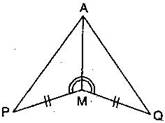Steps Reasons PM = QM {tex}\angle {/tex}PMA = {tex}\angle {/tex}QMA AM = AM {tex}\Delta {/tex}AMP {tex} \cong \Delta {/tex}AMQ __________ __________ __________ __________

 Steps Reasons PM = QM {tex}\angle {/tex}PMA = {tex}\angle {/tex}QMA AM = AM {tex}\Delta {/tex}AMP {tex} \cong \Delta {/tex}AMQ Given Given Common SAS congruence rule
###### Question 4.In {tex}\Delta {/tex}ABC, {tex}\angle {/tex}A = {tex}{30^ \circ },{/tex}{tex}\angle {/tex}B = {tex}40^\circ {/tex} and {tex}\angle {/tex}C = {tex}110^\circ .{/tex}

In {tex}\Delta {/tex}PQR, {tex}\angle {/tex}P = {tex}{30^ \circ },{/tex}{tex}\angle {/tex}Q = {tex}40^\circ {/tex} and {tex}\angle {/tex}R = {tex}110^\circ {/tex}.

A student says that {tex}\Delta {/tex}ABC {tex} \cong {/tex}{tex}\Delta {/tex}PQR by AAA congruence criterion. Is he justified? Why or why not?

No, because the two triangles with equal corresponding angles need not be congruent. In such a correspondence, one of them can be an enlarged copy of the other.

NCERT Solutions for Class 7 Maths Exercise 7.2

Question 5.In the figure, the two triangles are congruent. The corresponding parts are marked. We can write {tex}\Delta {/tex}RAT {tex} \cong {/tex} ?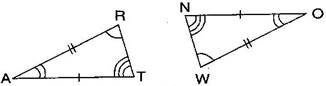In the figure, given two triangles are congruent. So, the corresponding parts are:

A {tex} \leftrightarrow {/tex} O, R {tex} \leftrightarrow {/tex} W, T {tex} \leftrightarrow {/tex} N.

We can write, {tex}\Delta {/tex}RAT {tex} \cong {/tex}{tex}\Delta {/tex}WON [By SAS congruence rule]

NCERT Solutions for Class 7 Maths Exercise 7.2

###### Question 6.Complete the congruence statement: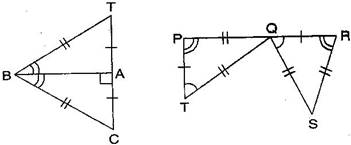{tex}\Delta {/tex}BCA {tex} \cong {/tex} ? {tex}\Delta {/tex}QRS {tex} \cong {/tex} ?

In {tex}\Delta {/tex}BAT and {tex}\Delta {/tex}BAC, given triangles are congruent so the corresponding parts are:

B {tex} \leftrightarrow {/tex} B, A {tex} \leftrightarrow {/tex} A, T {tex} \leftrightarrow {/tex} C

Thus, {tex}\Delta {/tex}BCA {tex} \cong {/tex}{tex}\Delta {/tex}BTA [By SSS congruence rule]

In {tex}\Delta {/tex}QRS and {tex}\Delta {/tex}TPQ, given triangles are congruent so the corresponding parts are:

P {tex} \leftrightarrow {/tex} R, T {tex} \leftrightarrow {/tex} Q, Q {tex} \leftrightarrow {/tex} S

Thus, {tex}\Delta {/tex}QRS {tex} \cong {/tex}{tex}\Delta {/tex}TPQ [By SSS congruence rule]

NCERT Solutions for Class 7 Maths Exercise 7.2

###### Question 7.In a squared sheet, draw two triangles of equal area such that:

(i) the triangles are congruent.

(ii) the triangles are not congruent.

What can you say about their perimeters?

In a squared sheet, draw {tex}\Delta {/tex}ABC and {tex}\Delta {/tex}PQR.

When two triangles have equal areas and

(i) these triangles are congruent, i.e., {tex}\Delta {/tex}ABC {tex} \cong {/tex}{tex}\Delta {/tex}PQR

[By SSS congruence rule]

Then, their perimeters are same because length of sides of first triangle are equal to the length of sides of another triangle by SSS congruence rule.

(ii) But, if the triangles are not congruent, then their perimeters are not same because lengths of sides of first triangle are not equal to the length of corresponding sides of another triangle.

###### Question 8.Draw a rough sketch of two triangles such that they have five pairs of congruent parts but still the triangles are not congruent.

Let us draw two triangles PQR and ABC.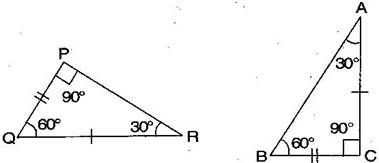All angles are equal, two sides are equal except one side. Hence, {tex}\Delta {/tex}PQR are not congruent to {tex}\Delta {/tex}ABC.

NCERT Solutions for Class 7 Maths Exercise 7.2

###### Question 9.If {tex}\Delta {/tex}ABC and {tex}\Delta {/tex}PQR are to be congruent, name one additional pair of corresponding parts. What criterion did you use?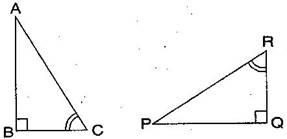{tex}\Delta {/tex}ABC and {tex}\Delta {/tex}PQR are congruent. Then one additional pair is {tex}\overline {{\text{BC}}} {/tex} = {tex}\overline {{\text{QR}}} .{/tex}

Given: {tex}\angle {/tex}B = {tex}\angle {/tex}Q = {tex}{90^ \circ }{/tex}

{tex}\angle {/tex}C = {tex}\angle {/tex}R

{tex}\overline {{\text{BC}}} {/tex} = {tex}\overline {{\text{QR}}} {/tex}

Therefore, {tex}\Delta {/tex}ABC {tex} \cong {/tex}{tex}\Delta {/tex}PQR [By ASA congruence rule]

NCERT Solutions for Class 7 Maths Exercise 7.2

###### Question 10.Explain, why {tex}\Delta {/tex}ABC {tex} \cong {/tex}{tex}\Delta {/tex}FED.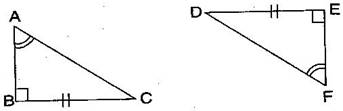Given: {tex}\angle {/tex}A = {tex}\angle {/tex}F, BC = ED, {tex}\angle {/tex}B = {tex}\angle {/tex}E

In {tex}\Delta {/tex}ABC and {tex}\Delta {/tex}FED,

{tex}\angle {/tex}B = {tex}\angle {/tex}E = {tex}{90^ \circ }{/tex}

{tex}\angle {/tex}A = {tex}\angle {/tex}F

BC = ED

Therefore, {tex}\Delta {/tex}ABC {tex} \cong {/tex}{tex}\Delta {/tex}FED [By RHS congruence rule]

## NCERT Solutions for Class 7 Maths Exercise 7.2

NCERT Solutions Class 7 Maths PDF (Download) Free from myCBSEguide app and myCBSEguide website. Ncert solution class 7 Maths includes text book solutions from Class 7 Maths Book . NCERT Solutions for CBSE Class 7 Maths have total 15 chapters. 7 Maths NCERT Solutions in PDF for free Download on our website. Ncert Maths class 7 solutions PDF and Maths ncert class 7 PDF solutions with latest modifications and as per the latest CBSE syllabus are only available in myCBSEguide.

## CBSE app for Students

To download NCERT Solutions for Class 7 Maths, Social Science Computer Science, Home Science, Hindi English, Maths Science do check myCBSEguide app or website. myCBSEguide provides sample papers with solution, test papers for chapter-wise practice, NCERT solutions, NCERT Exemplar solutions, quick revision notes for ready reference, CBSE guess papers and CBSE important question papers. Sample Paper all are made available through the best app for CBSE students and myCBSEguide website.Test Generator

Create question paper PDF and online tests with your own name & logo in minutes.myCBSEguide

Question Bank, Mock Tests, Exam Papers, NCERT Solutions, Sample Papers, Notes

### 10 thoughts on “NCERT Solutions for Class 7 Maths Exercise 7.2”

1. but if we have to show that all the sides and the angles are not equal then what should we do .

2. It’s awesome

3. Sanaa do sirf lekha mat kro

4. Ans 8 is wrong

5. Help full me

6. In question 8 i have a doubt.

7. I have a dout in question 7

8. Its really helpful math solutions

9. You are so helpful to us

10. You are so helpful to us